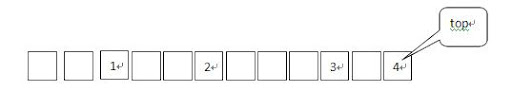# PKU1988 Cube Stacking 解题报告

February 10, 2010 / ACM, 解题报告

1.Ｍ　Ｘ　Ｙ　：将Ｘ所在的堆叠在Ｙ所在的堆上面，保证Ｘ和Ｙ不在同一堆。

2.Ｃ　Ｘ　：计算在Ｘ所在的堆中在Ｘ下方的有多少立方块，并输出。

Pre[ MAX ]：每个立方块所在堆中最上面的立方块的标号

Up[MAX]：在Ｘ立方块所在的堆中在Ｘ上面的立方块个数

Down[MAX]：在每个堆中最上面的立方块下面的立方块个数

1.初始化

Pre[ i ] = i; i = {1…MAX} , 每堆只有一个立方块，即立方块所在堆最顶上立方块是其本身

Up[ i ] = Down[ i ] = 0; i = {1…MAX}

2.执行M操作Up[ 1 ] = 3, Up[ 2 ] = 4, Up[ 3 ] = 2,Up[ 4 ] = 0;

``````int find( int x ){
int r = x, q, h = 0;

while ( pre[ r ] != -1 ){
h += Up[ r ];    //对每一组方块长度进行叠加
r = pre[ r ];
}

//退出循环时，r就是x所在堆最上边的方块，h是x上边的方块数
while ( x != r ){
h -= Up[ x ];//将已计算过的方块数目减去
Up[ x ] += h;//更新每一方块上面的方块数
q = pre[ x ];
pre[ x ] = r;
x = q;
}

return r;
}``````

3.执行C操作